# Control of Intrachannel Nonlinear Effects

It is clear from the pseudo-linear lightwave systems tutorial that intrachannel XPM and FWM can limit the performance of a pseudo-linear system. Both of these effects may occur even for systems making use of dispersion-managed (DM) solitons because pulses overlap partially during each map period. It is thus important to find ways to reduce their impact through a proper system design. In this tutorial, we focus on several such schemes.

#### 1. Optimization of Dispersion Maps

The design of any lightwave system requires an appropriate dispersion map. There are two main choices. In one, dispersion accumulates along most of the link length and is compensated using DCFs only at the transmitter and receiver ends (pre- and post-compensation). In the other, dispersion is compensated periodically along the link (in-line compensation), either completely or partially. In the later situation, DCFs may be used at the two ends for compensating the residual dispersion. Both types of dispersion maps have been used for 40-Gb/s expriments.

In a 1998 experiment, dispersion accumulated over a 150-km link with D = 2.3 ps/(km-nm) was fully compensated through pre- and post-compensation. Pre-compensation worked only if the launch power was below 5 dBm. Much higher powers could be launched (up to 12 dBm) in the case of post-compensation, while keeping the penalty below 0.5 dB. A similar dispersion map was employed in a 2000 experiment in which a 40-Gb/s signal was transmitted over 800 km by amplifying it periodically every 80 km. This experiment used standard fibers and compensated the entire accumulated dispersion (da > 12 ps/nm) at the receiver end. It also operated in the pseudo-linear regime as it employed 2.5-ps pulses to generate the 40-Gb/s bit stream. The amplifier spacing was increased to 120 km in a later experiment. Figure (a) below shows the measured Q factor as a function of launched power after 3, 4, 5, and 6 amplifier. At a distance of 600 km, Q2 exceeded 15.6 dB (a value needed to keep the BER below 10-9) over a large power range from 4 to 11 dBm. However, when link length was 720 km, this value of Q2 was obtained only for an input power level close to 8 dBm. As seen in figure (b) below, much longer distances could be realized by reducing the spacing between amplifiers to 80 km.A recirculating-loop configuration is often used in practice for 40-Gb/s experiments employing periodic dispersion maps. In an experiment operating in the pseudo-linear regime, dispersion accumulated over 100 km of dispersion-shifted fiber was compensated using a higher-order-mode DCF (see the dispersion compensating fiber - DCF tutorial). The span loss of 22 dB was compensated using a hybrid amplification scheme, with 15 dB of distributed Raman gain realized through backward pumping. In this experiment, 40-Gb/s data could be transmitted over 1,700 km while maintaining a BER below 10-9. The same approach was used in another loop experiment in which loop length was 75 km, and the DCF did not fully compensate its dispersion. The residual dispersion of -1.4 ps/nm per round trip was compensated outside the loop, just before the receiver. The DCF location was changed within the loop to simulate the pre- and post-compensation situations. In general, post-compensation provided better performance in the case of the RZ format. The intrachannel four-wave mixing (FWM) theory of the pseudo-linear lightwave systems tutorial shows that the power transferred to ghost pulses is sensitive to the amount of precompensation. In this experiment, the transmission distance was limited to about 700 km.

Several 40-Gb/s experiments designed the dispersion map such that the system operated in the DM-soliton regime (refer to the DM-soliton tutorial). In one set of experiments, the average GVD of a 106-km-long recirculating loop was varied to optimize system performance. It was found that the system could be operated over more than 1,500 km by adjusting the launched power as long as the average dispersion was anomalous in the range of 0 to 0.1 ps/(km-nm). Much better performance was realized in another experiment in which a 40-Gb/s signal in the form of DM solitons could be transmitted over 6,400 km. In general, soliton systems are found to be limited by XPM-induced timing jitter, whereas pseudo-linear systems are limited by FWM-generated ghost pulses.

The optimization of a dispersion map is not a trivial task as it involves varying a large number of design parameters (lengths and dispersion of individual fibers used to make the map, the amount of pre- and post-compensation employed, pulse width, etc.) for a given set of system parameters (such as bit rate, link length, amplifier spacing). Extensive numerical simulations reveal several interesting features. Both the pseudo-linear and DM-soliton regimes can be used to design 40-Gb/s systems, but the dispersion map for them is generally quite different. When fiber dispersion is relatively small along most the link [D < 4 ps/(km-nm)], the soliton regime works best with an RZ duty cycle near 50% and requires some residual dispersion per map period. The system can also be designed in the pseudo-linear regime if the duty cycle is reduced to below 30% and the amount of pre- and post-compensation is suitably optimized. In contrast, when dispersion is large along most of the link (as is the case when standard single-mode fibers are employed), operation in the pseudo-linear regime may be more desirable for designing a 40-Gb/s system. Even though a single-channel soliton system may operate at 40-Gb/s over longer lengths under such conditions, the pseudo-linear regime is often preferred for WDM systems.

It turns out that the intrachannel nonlinear effects can be controlled with a suitable choice of the dispersion map. As discussed earlier, XPM-induced timing jitter can be reduced considerably by making the dispersion map symmetric. In fact, as seen in the figure below, both timing and amplitude jitters can be reduced by making the accumulated dispersion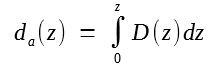symmetric over the link such that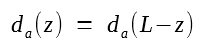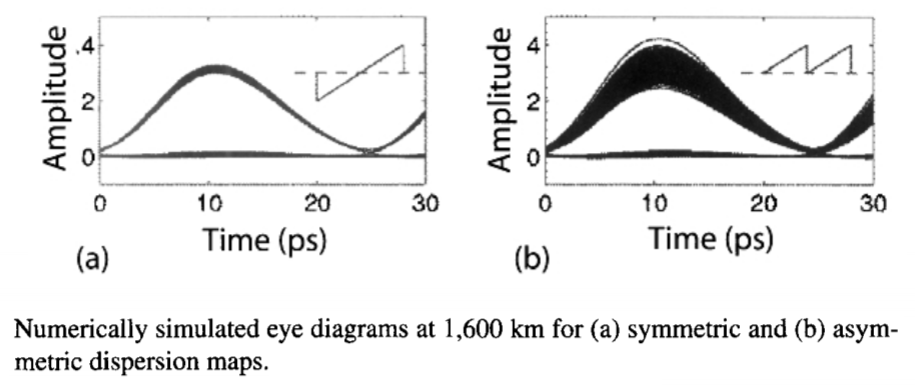In practice, this can be realized by compensating 50% of dispersion at the transmitter end and the remaining 50% at the receiver end. Such a map was used for numerical simulations shown in the figure above for which 2.5-ps Gaussian pulses with a 25-ps bit slot were propagated over 1,600 km of standard fiber with D = 17 ps/(km-nm). The following equation can be used to understand why jitter may be reduced for symmetric maps by noting that the limits of integration should be changed from -L/2 to L/2 for such maps.As discussed earlier, timing jitter results from XPM-induced frequency shifts that cancel for a symmetric map. Indeed, timing jitter would vanish under such conditions if p(z) = 1 in the equation above. The residual jitter seen in the figure above is due to variations in the average power of the signal along the link induced by a lumped amplification scheme. The amplitude jitter depends on the relative phase between the existing pulse in a bit slot and the nonlinear perturbation ΔU produced in that bit slot by other neighboring pulses. The in-phase part of the perturbation nearly vanishes for symmetric maps, resulting in reduced amplitude fluctuations.

If a periodic dispersion map is employed with two fiber sections of equal lengths but opposite dispersions, the jitter reduction can be realized by reversing the two fibers in every alternate map period. The following figure shows the reduction realized in the amplitude and timing jitters at three power levels with such symmetrization of the dispersion map. The calculated eye-opening penalty (EOP) is also shown. Each span employs two 40-km fiber sections with D = ±17 ps/(km-nm), α = 0.2 dB/km, and γ = 1.1 W-1/km. In the case of a conventional periodic dispersion map, both the amplitude and timing jitters increase linearly with the link length and become so large that the EOP exceeds 4 dB after 700 km for a launched power of 6 dBm. In contrast, when the map is made symmetric, jitter accumulated over one span is cancelled to a large extent in the following span. As a result, the net timing jitter oscillates and increases much more slowly for symmetric maps. With a simple change that involves only flipping the fibers in alternate span, the same system can operate over more than 1,200 km with negligible penalty. The length of two fiber sections in the dispersion maps does not need to be equal for the cancellation of jitter to occur. The concept of scaled translation symmetry can be used to show that both the amplitude and timing jitters can be reduced for a wide variety of maps, even when variations in the average power are not symmetric around the midpoint.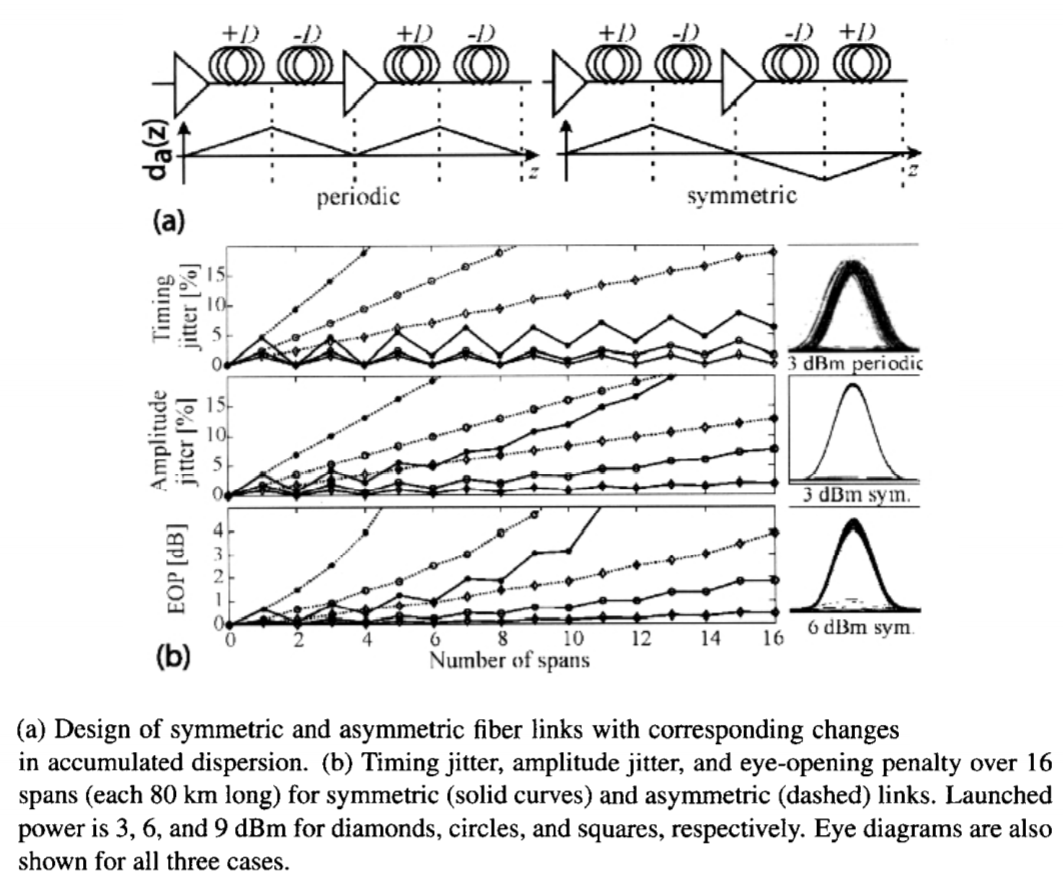The use of optical phase conjugation can also reduce the intrachannel nonlinear effects. As discussed in the optical phase conjugation tutorial, such a device is equivalent to reversing the sign of the dispersion parameters of all fiber sections in the second half of the link. System-level experiments have confirmed that phase conjugation can reduce the impact of intrachannel nonlinear effects considerably.

#### 2. Phase-Alternation Techniques

As the nonlinear perturbation in the ΔUjkl equation above depends on the phase of pulses generating it, the input phase of optical pulses forming the bit stream can be used to control the intrachannel nonlinear effects. The basic idea consists of introducing a relative phase shift between any two neighboring bits, resulting in a modulation format referred to as the alternate-phase RZ (AP-RZ) format. Several other formats, such as carrier-suppressed RZ (CSRZ), duobinary RZ, RZ-DPSK, and alternate-mark-inversion (AMI) RZ, can also be employed. The last format was used in a 2003 experiment to transmit a 40-Gb/s signal over 2,000 km. The improvement in the Q factor was typically less than 1 dB when compared with the standard RZ format.

The important question is which phase-alternation technique is the optimum choice for suppressing the intrachannel nonlinear effects. Since ghost pulses generated through intrachannel FWM are often the limiting factor for pseudo-linear systems, one can ask which technique reduces their amplitude most. Four modulation formats were compared in a numerical study, whose results are shown in the figure below.The Q factor is plotted for a 40-Gb/s system at a distance of 1000 km for two dispersion maps with a 100-km map period. The map (a) consists of three sections such that D = 19 ps/(km-nm) for the first and third sections (each 30 km long) but D = -28 ps/(km-nm) for the 40-km-long middle section. The map (b) employs 100 km of standard fiber with D = 17 ps/(km-nm) whose dispersion is compensated using DCFs. The duty cycle is typically 66% for the CSRZ format but only 33% for the standard RZ format. As seen in the figure, both the DPSK and AMI formats provide better performance compared with RZ and CSRZ formats. This can be understood by noting that the amplitude of ghost pulses generated through various combinations of the subscripts j, k, and l in the equation above depends on the phase of neighboring bits, among other things. The amount of improvement realized with the use of DPSK and AP-RZ formats depends on the launched power. In general, more power can be launched when phase-alternation techniques are employed.

The CSRZ format is an example of the AP-RZ format for which the phase difference δφ between two neighboring bits is fixed at a value of π. Clearly, one can choose δφ in the range of 0 to π, although the optical carrier may then not be suppressed completely. A numerical study shows that the optimum value of δφ is close to π/2, as this choice minimizes the buildup of ghost pulses generated through intrachannel FWM. The figure below shows the standard deviation of power in 0 bits (calculated numerically) as the function of distance for a 40-Gb/s signal with 25% duty-cycle pulses. The dispersion map consists of 60 km of standard fiber, followed by 12 km of a DCF. As expected from the theory of pseudo-linear lightwave systems tutorial, power in 0 bits increases rapidly in a quadratic fashion for a standard RZ signal (δφ = 0). The growth is reduced slightly in the case of the CSRZ format (δφ = π). However, it is suppressed by a large amount for δφ = π/2. Experimental results support this conclusion.#### 3. Polarization Bit Interleaving

Another technique for controlling the intrachannel nonlinear effects alternates the polarization of neighboring bits in an RZ signal. It makes use of the fact that both the XPM and FWM processes depend on the state of polarization (SOP) of the interacting waves. The technique of polarization bit interleaving was first used in 1991 for reducing interaction between neighboring solitons. In another approach, used commonly for increasing the spectral efficiency of WDM systems, neighboring channels are orthogonally polarized. However, this scheme is quite different from the one considered in this section in which neighboring bits of a single channel are orthogonally polarized through time-domain interleaving.

The following figure shows two schemes that can be used for polarization alternation on a bit by bit basis. In both of them, a pulse carver is used to create an uncoded train of RZ pulses at the bit rate rate. In scheme (a), a phase modulator operating at half the bit rate first imposes a phase shift on this pulse train, which is split into its orthogonally polarized components that are combined back after one bit delay. A data modulator then codes the RZ signal. In scheme (b), in contrast, the pulse train is first coded with the data. It is then split into its orthogonally polarized components that are combined back after a phase modulator first imposes a phase shift on one of the components. The second scheme is much easier to implement in practice. The signal spectrum is affected considerably with polarization alternation. In particular, the spectra of orthogonally polarized components have side bands spaced apart by the bit rate B, but they are shifted by B/2.Considerable reduction in the power level of ghost pulses generated through intrachannel FWM was observed in a 40-Gb/s recirculating-loop experiment. The loop included four spans of 82.5 km, each span formed with 70 km or so of standard fiber followed with a DCF that left a residual dispersion of 40 ps/nm per span. Backward Raman pumping was used for compensating span losses in a distributed fashion. The following figure shows the measured BER as a function of launch power after a distance of 2,000 km (six round trips in the loop). Four curves correspond to four different modulation formats. The duty cycle of RZ and CSRZ bit streams are 33% and 66%, respectively.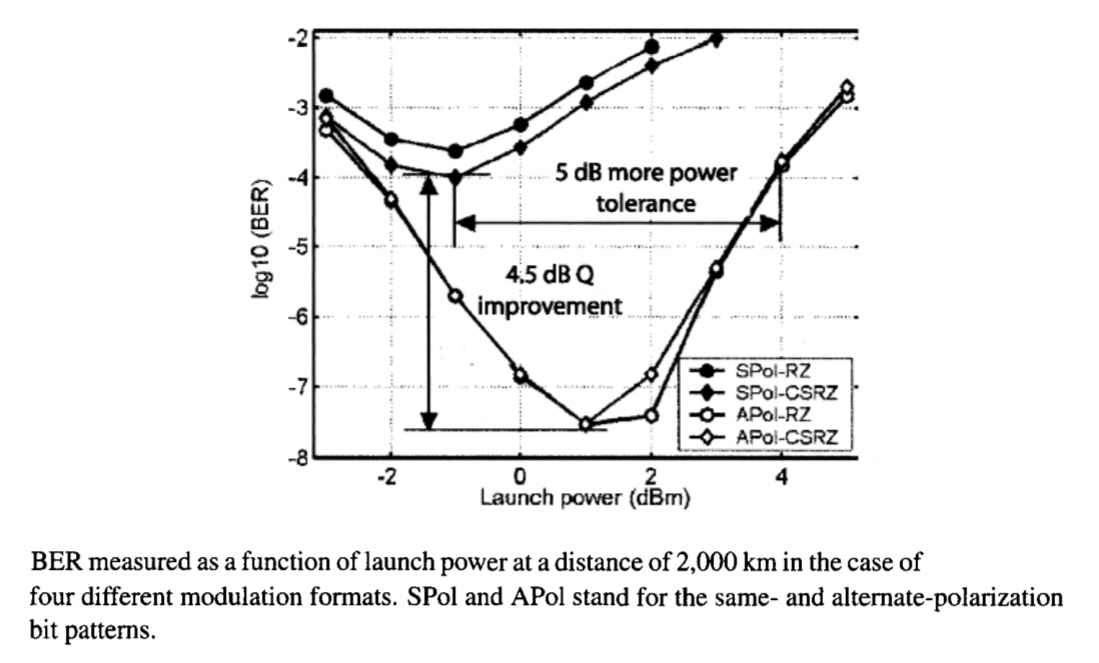Several features of the figure above are noteworthy. First, the minimum BER was ≥ 10-4 for both the RZ and CSRZ formats when all bits had the same SOP and it was realized at a relatively low value of launch power. When the polarization-alternation technique was implemented, the BER improved considerably and its minimum value occurred at a higher power level (about 1 dBm). These results indicate that the Q2 factor improves by 4.5 dB when neighboring bits are orthogonally polarized and can be understood as follows. with polarization alternation, intrachannel nonlinear impairments are reduced significantly and lead to a much lower BER for the RZ format, as evident from the figure. Since the duty cycle was 66% for the CSRZ signal, and each 1 bit had a wider pulse with, its performance was slightly lower than the RZ signal (with a 33% duty cycle) under the same operating conditions. The main conclusion is that the use of polarization alternation in the time domain helps considerably to reduce the intrachannel nonlinear effects in high-speed lightwave systems operating at bit rates of 40 Gb/s or more. A low-duty RZ format is often the optimum choice for such systems so that the system operates in the pseudo-linear regime.